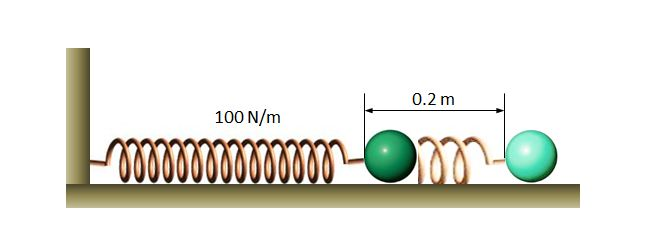# Oscillation of a springA spring with spring constant $100$ N/m is fixed on the left wall in the above diagram, with an iron ball tied to other end. If we pull the iron ball by $0.2$ m to the right, and then release it, the motion of the iron ball will be a harmonic oscillation. If the mass of the iron ball is $1$ kg and the floor is frictionless, what is the period of the harmonic oscillation of the iron ball?

×